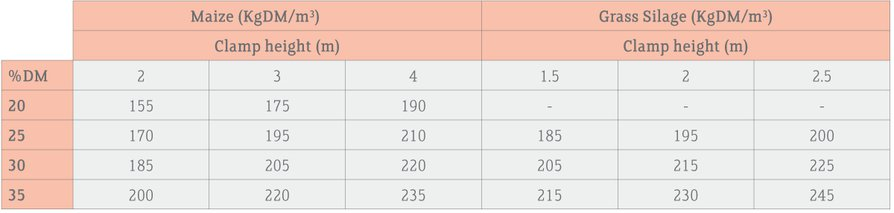# How we calculated your clamp silage stocks

### Example calculation based on the following:

• Clamp silage dimensions (L x W x H) = 40 x 15 x 2 metres
• Silage DM = 30%
• 100 cows eating 35 kg fresh silage per cow per day

Step 1: Calculate the volume (m3) of silage dry matter in the clamp.

Silage fresh matter = 40 x 15 x 2 = 1,200 m3.

Step 2: Convert this into kg silage DM using the table.For a 2 metre high clamp of 30% DM grass silage the average DM density is 185 kgDM/m3.

Clamp contains 1,200 x 185 =222,000 kg DM = 222 tonnes DM.

Step 3: Calculate daily silage DM requirements for the herd:

= Number of cows x daily fresh silage intake per cow x 30/100
= 100 x 35 x 30/100 = 1,050 kg = 1.05 t silage DM per day required.

Step 4: Calculate how many days of silage you have in stock.

= tonnes silage DM/silage DM requirements per day = 222/1.05 = 211 days